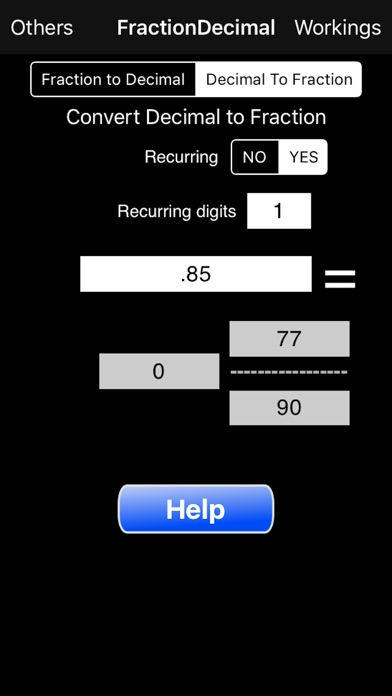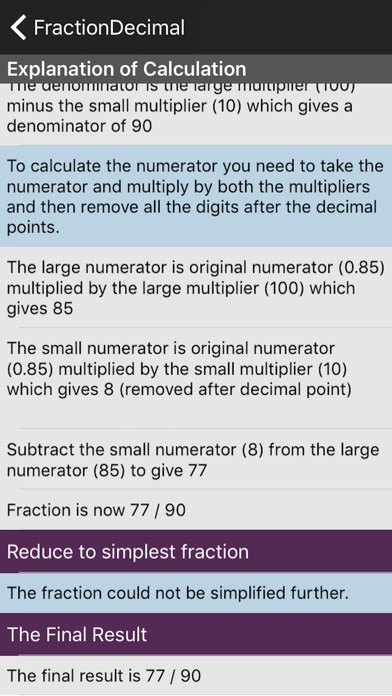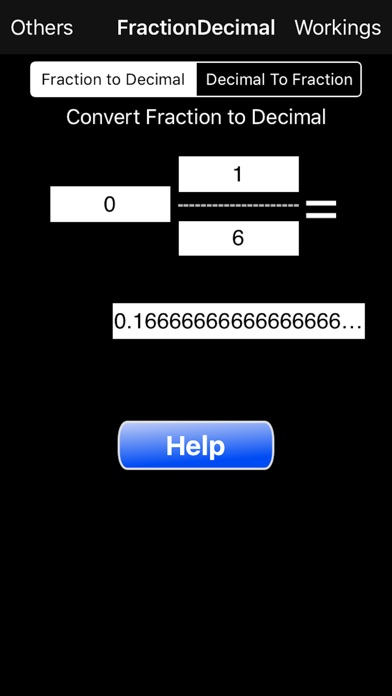Fractions/Decimals/Fractions

iOS UniversalEducation

\$0.99

Do you need to convert fractions into decimals or decimals into fractions? Can't quite remember how to do it? Well this App is for you. Not only does it do the calculations for you but it also provides comprehensive explanations as to how it was done so that you can not only know the answer but understand how it was arrived at. While some calculations are easy enough converting a recurring decimal into a fraction is not. You need to know how it is done and this App explains how step by step.

The App can take a fraction and convert it into its decimal equivalent and also provide a page that explains how the answer was arrived at. You can even see whether the decimal is recurring or not. Just type in the fraction in the three boxes provided and the decimal equivalent will be shown. If the answer ends in three '...' then the number is recurring.

The App can also take a decimal and convert it into its equivalent fraction providing a page that explains how the answer was arrived at. The decimal number can either be specified as recurring or not and if it is how many digits are recurring.

Some examples,

0.3 not recurring is 3/10

0.3 with one digit recurring is 1/3 (specify recurring Yes and recurring digits 1)

0.311 not recurring is 311/1000

0.301 with one digit recurring is 271/900 (specify recurring Yes and recurring digits 1)

0.301 with two digits recurring is 149/495 (specify recurring Yes and recurring digits 2)

0.142857142857 with six digits recurring is 1/7 (specify recurring Yes and recurring digits 6)

In the final example the 6 recurring digits are specified twice but this does not matter to the App. The excess digits are carefully removed and the calculation done with 0.142857

New Version: To those of you who have purchased the Fractions Calculator functionality. You can now have negative fractions. Either specify the whole number as negative or if zero just put a minus sign.

•••